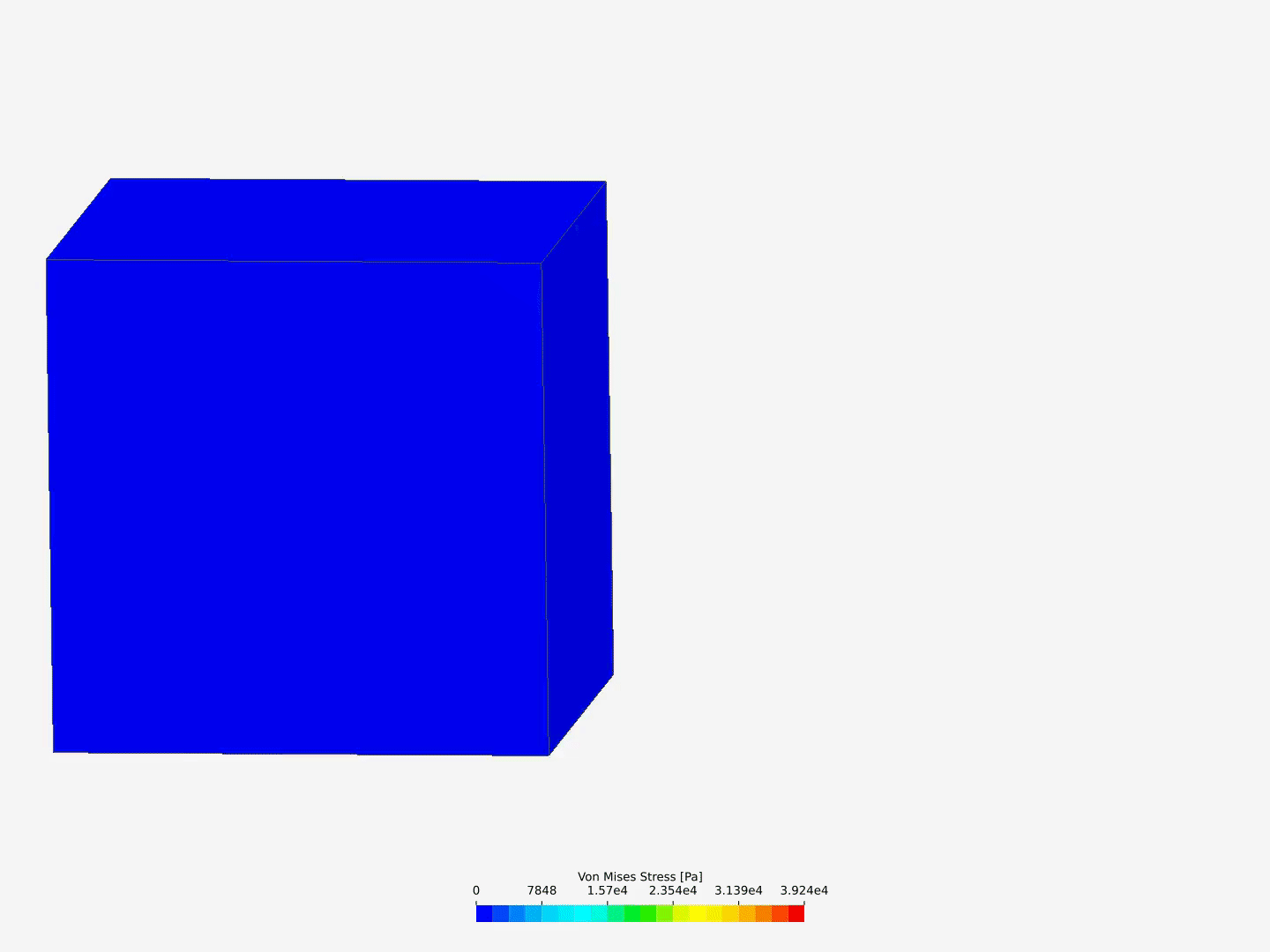# Hyperealsticity - Neo-Hookean material model

I am trying to make a simple example of a nonlinear static solid mechanics model. For this I used a unit cubid [0,1]^3. At x==0 the cuboid is fully constraint (u=v=w=0 displacement) and at x==1 I apply a force of 1N in the positive x direction. The force is ramped via 1t(t<1)+1*(t>=1). The material is supposed to be something like Rubber, so I choose a hyperelastic neo-hookean material model. The material parameter C10 (=mu/2) is set to 0.2 MPa and the material parameter D1 (=2/K) is set to 1e-9 Pa.

The solver computes some steps but then gives up.

The project is here.

I have seen this hyperelastic example but I was not able to adopt it to my case. What surprises me in this given example that the stain is constant. Also I would nice to be able to see both the original and the deformed shape in one plot.

Hi @ruebenko ,
I think your applied displacement is just too high.
You have a cube of 1mm edge length and apply a displacement with a formula of DX = “t” [m], so for the 1 s runtime, you have 1 m displacement.
I see the solver was able to successfully compute the solution until about 2.7 mm.

I added a new simulation run here with a displacement formula of DX = “0.001*t”, so you will have 1mm displacement at the end of your 1s Simulation interval: SimScale Login

Results look reasonable at least, but the asymmetric stresses show that you should probably refine the mesh to get accurate results:I also changed the residual back to relative and the “Linear system relative residual” to “-1” (maning deactivated) as its not needed for a nonlinear case where convergence is already ensured within the Newton method itself.
For a higher deformation you can either increase the “Simulation interval” or adjust the fixed displacement formula.

Best,
Richard

1 Like

Thanks @rszoeke . Much appreciated. I totally missed the scale of the geometry. As a suggestion for a future development, perhaps the scale of the object can be mentioned in the Geometry section. That would have helped me.

1 Like